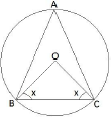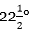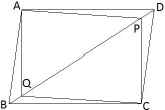# UP Board Important Questions for Class 9 Maths

Maths is all about practicing. The more you practice the more your concept will be cleared. Only just reading the concepts & mugging up the formulas will not going to prepare you for the UP Board class 9 Maths exam. You need to practice a lot score gain good marks in the Mathematics subject of UP Board Class 9 annual exam. Therefore, to make you prepare in a better away here we have provided the set of UP Board Important Questions for Class 9 Maths.

### Why Solve UP Board Important Questions for Class 9 Maths?

UP Board Important Questions for Class 9 Maths Gives you a competitive edge. Here are a few points that highlight the importance of solving UP Board Class 9 Maths questions.

• This set of Class 9 Maths Important Questions of UP Board covers the important topics of mathematics.
• These UP Board Important Questions for Class 9 Maths are being prepared by the subject experts.
• You could able to manage the time efficiently during the actual exam.
• It will improve your performance in the exam.
• By practicing these important questions, maths formulas will be in your fingertips.

## UP Board Important Questions for Class 9 Maths

The Questions number from 1 to 8 below carries 3 marks each:
Q1) Solve the equation 3 (𝑥+2) = 2 (2𝑥 − 1) and represent the solution on: i. the Number Line. ii. the Cartesian Plane

Q2) ABCD is a parallelogram. The bisector of angle A also bisects BC at X. Prove that AD = 2AB.

Q3) Angles of a quadrilateral are in ratio 2:4:5:7. Find all the angles.

Q4) Prove that the median of a triangle divides it into two triangles of equal area.

Q5) In the given figure, O is the center of the circle. Prove that ∠𝑂𝐵𝐶+∠𝐵𝐴𝐶=90°Q6) Construct an angle ofusing scale and compass only.

Q7) A solid cube of side 12 cm is cut into eight cubes of equal volume. What will be the side of the new cube?

Q8) Eleven bags of wheat flour, each marked 5 kg, actually contained the following weights of flour (in kg). 4.97, 5.05, 5.08, 5.03, 5.00, 5.06, 5.08, 4.98, 5.04, 5.07, 5.00. Find the probability that any of these bags chosen at random contains more than 5 kg of flour.
The Questions number from 9 to 20 below carries 4 marks each:

Q9) Let the cost of a pen and a pencil be ‘x’ and ‘y’ respectively. A girl pays Rs 16 for 2 Pens and 3 Pencils. Write the given data in the form of a linear equation in two variables. Also, represent it graphically.

Q10) Prove that the quadrilateral formed (if possible) by the internal angle bisectors of any quadrilateral is cyclic.

Q11) In the figure, ABCD is a parallelogram and P, Q are the points on the diagonal BD such that BQ = DP. Show that APCQ is a parallelogram.Q12) Draw the graph of linear equation x = 4 and y = 5. Find the area formed by the two graphs and the axes.

Q13) A metal pipe is 77 cm long. The inner diameter of a cross-section is 4 cm, the outer diameter being 4.4 cm. Find its total surface area.

Q14) Construct a Δ𝑋𝑌𝑍 in which ∠𝑌=60°,∠𝑍=45° 𝑎𝑛𝑑 𝑋𝑌+𝑌𝑍+𝑍𝑋=11 𝑐𝑚. Also, write the steps of construction.

Q15) In a society, 6 children out of 28 did not participate in the campaign ‘Save Energy’. Find the probability that a child selected at random. i. participated in the Campaign. ii. did not participate in the Campaign. Which values of children are depicted here?

Q16) A cylindrical pillar is 50 cm in diameter and 3.5 m in height. Find the cost of painting the curved surface of the pillar at the rate of Rs 12.50 per meter square.

Q17) How many liters of milk can a hemispherical bowl of diameter 10.5 cm hold?

Q18) Which social networking site is more beneficial in learning mathematics and how?

Q19) In a parallelogram ABCD, if ∠𝐴= 2𝑥+25 ° 𝑎𝑛𝑑 ∠𝐵= 3𝑥−5 °, find the value of x.

Q20) Find the points on the graph of the linear equation 3𝑥+5𝑦=15, where it cuts the x-axis and y-axis.

Having a good foundation in class 9 maths will make it easier for you to score good marks in Class 10. However, one should not forget to solve more up board 10th model paper to get more practice.

Learn & Practice more on different topics to excel in UP Board Class 9 Maths exam. Stay tuned for more updates on UP Board exam and study materials like